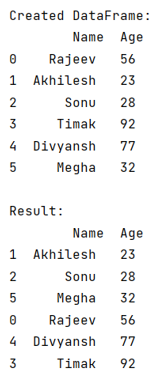# How to sort a dataFrame in python pandas by two or more columns?

Given a Pandas DataFrame, we have to sort a dataFrame by two or more columns.
Submitted by Pranit Sharma, on July 28, 2022

Pandas is a special tool that allows us to perform complex manipulations of data effectively and efficiently. Inside pandas, we mostly deal with a dataset in the form of DataFrame. DataFrames are 2-dimensional data structures in pandas. DataFrames consist of rows, columns, and data.

Sorting refers to rearranging a series or a sequence in a particular fashion (ascending, descending, or in any specific pattern). Sorting in pandas DataFrame is required for effective analysis of the data. Pandas allow us to sort the DataFrame using pandas.DataFrame.sort_values() method.

Syntax:

```DataFrame.sort_values(
by,
axis=0,
ascending=True,
inplace=False,
kind='quicksort',
na_position='last',
ignore_index=False,
key=None
)
```

Parameters:

• by: name of sequence or column by which it should sort.
• axis: Column to be sorted.(0 or 'axis' 1 or 'column') by default its 0 (column number)
• There are some other parameters like: Ascending, inplace, kind, etc.

Let us understand with the help of an example,

## Python code to sort a dataFrame in pandas by two or more columns

```# Import pandas package
import pandas as pd

# import numpy package
import numpy as np

# Creating a dictionary
d = {
'Name': ['Rajeev', 'Akhilesh', 'Sonu', 'Timak', 'Divyansh', 'Megha'],
'Age': [56, 23, 28, 92, 77, 32]
}

# Creating a Dataframe
df = pd.DataFrame(d)

# Dispaly the dataframe
print('Created DataFrame:\n',df,"\n")

# Using the sorting function
result = df.sort_values(by=['Age'], ascending=True)

# Display result
print('Result:\n',result)
```

Output:What's New

Top Interview Coding Problems/Challenges!

Languages: » C » C++ » C++ STL » Java » Data Structure » C#.Net » Android » Kotlin » SQL
Web Technologies: » PHP » Python » JavaScript » CSS » Ajax » Node.js » Web programming/HTML
Solved programs: » C » C++ » DS » Java » C#
Aptitude que. & ans.: » C » C++ » Java » DBMS
Interview que. & ans.: » C » Embedded C » Java » SEO » HR
CS Subjects: » CS Basics » O.S. » Networks » DBMS » Embedded Systems » Cloud Computing
» Machine learning » CS Organizations » Linux » DOS
More: » Articles » Puzzles » News/Updates